Science Journal of Applied Mathematics and Statistics
Volume 4, Issue 6, December 2016, Pages: 289-297

Modeling Loan Defaults in Kenya Banks as a Rare Event Using the Generalized Extreme Value Regression Model

Stephen Muthii Wanjohi, Anthony Gichuhi Waititu, Anthony Kibira Wanjoya

Department Statistics and Actuarial Science, Jomo Kenyatta University of Agriculture and Technology, Nairobi, Kenya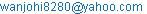(S. M. Wanjohi)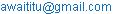(A. G. Waititu)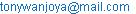(A. K. Wanjoya)

Stephen Muthii Wanjohi, Anthony Gichuhi Waititu, Anthony Kibira Wanjoya. Modeling Loan Defaults in Kenya Banks as a Rare Event Using the Generalized Extreme Value Regression Model. Science Journal of Applied Mathematics and Statistics. Vol. 4, No. 6, 2016, pp. 289-297. doi: 10.11648/j.sjams.20160406.17

Received: October 4, 2016; Accepted: October 25, 2016; Published: November 16, 2016

Abstract: Extreme value theory is the study of extremal properties of random processes, it models and measures events that occur with little probability. The extreme value theory is a robust framework to analyze the tail behavior of distributions. It has been applied extensively in hydrology, climatology, insurance and finance industry. The information of probability of customer default is very useful while analyzing the credit risks in banks. Logistic regression model has been used extensively to model the probability of loan defaults. However, it has some limitations when it comes to modeling rare events, for example, the underestimation of the default probability which could be very risky for the bank. The second limitation/drawback is that the logit link is symmetric about 0.5, this means that the response curve п(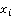) approaches one at the same rate it approaches zero. To overcome these limitations the study sought to implement regression method for binary data based on extreme value theory. The objective of the study was to model loan defaults in Kenya banks using the GEV regression model. The results of GEV were compared with the results of the logistic regression model. The study found out for rare events such as loan defaults the GEV performed better than the logistic regression model. As the percentage of defaulters in a sample became smaller the GEV model to identify defaults improves whereas the logistic regression model becomes poorer.

Keywords: Logistic, Generalized Extreme Value Regression, Extreme Value Theory, Confusion Matrix

1. Introduction and Literature Review

1.1. Background of Study

The likelihood that a bank loan will default is of interest to both regulators and investors. Under the Basel regulatory guidelines, a bank must hold capital in proportion to the riskless of its assets. The probability of default is the primary determinant of riskless on loan. Investor, in turn, prices a loan in the secondary market based on its normal cash flow, which again depends on the default probability.

Credit risk forecasting is one of the most studied topics in modern finance, as the bank regulation has made increasing use of external and internal credit rating (Basel Committee of banking supervision )

The Basel capital accord encourages financial institutions to develop and promote financial management systems. As a result, banks are interested in obtaining a more objective rating of loan portfolios.

High levels of indebtedness imply a greater incident of default and increasing the risk of lenders.

Since unsecured personal loans policies change rapidly, stringent measures and developing an efficient portfolio management strategy are important objectives of the banks. Banks consequently devote many resources to developing internal risk models. Once accurate credit risk models have been developed banks will be able to identify loans that have a lower probability of default.

In finance, the default is a failure to meet the legal obligation (or conditions) of a loan.eg when a home buyer fails to make a mortgage payment.

Most significant events in several areas are rare events. These include economics, finance, medicine, and epidemiology. In economics and finance, some critical areas of applications of extreme value theory and the rare event methodology are credit risk, value at risk and finance strategy of risk management (Embrechts et al )

Extreme Value Theory (E.V.T) is the theory of modeling and measuring events that occur with little probability.

In EVT theory, there are two main approaches with their strengths and weaknesses. The first one is based on modeling the maximum of a sample called the upper order statistics over a period.

The second relies on modeling excess values of a sample over a threshold within a period

The three families of the extreme value distributions can be nested into a single parameter representation as shown by Jenkison  and Von Mises .

This representation is known as the Generalized Extreme Value (GEV) distribution.

1.2. Models Previously Used to Model the Likelihood of Default

Altman  used the Z-score formula for predicting bankruptcy. The formula was used to predict the probability that a firm will go into bankruptcy within two years. The Z-score was also used to predict corperate defaults. The Z-score uses multiple corporate income and balance sheet values to measure the financial health of a company. The z-score is a Linear combination of five common business ratios; which are weighted by coefficients. He applied the statistical method of discriminant analysis to a data set of publicly held manufacturers

Lenntand Golet  in his paper (article) he focused on the symmetric binary choice models also known as conditional probability models. He sought to know whether asymmetric binary choice models, based on extreme value theory, can explain bankruptcy better.

Anatoly et al . In the study of the probability of default models of Russian banks, they used binary choice models to estimate the probability of default. They also found out that preliminary expert clustering or automatic clustering improves the predictive power of the models.

Mday Rajan et al.  In their work they focused on the statistical default models and incentives. They argued that a purely statistical model ignore the idea that a change in the incentives of agents who generate the data may change the very nature of data, their work tried to critique on statistical models that naively collaborate on historical data without modeling the agent behavior that produces these data.

Andrea Puth Coravos,  He focused on measuring the livelihood of small business loan default. Using small business loan portfolio data, he identified the specific borrower, lender and loan characteristics and changes in economic conditions that increase the likelihood of default. His results laid the foundation for an in-house, and it is scoring model.

Peter Croshie,  He focused his work on modeling default risk that it is the uncertainty surrounding a firm‘s ability to service its debts and obligations.

Alexander  His study focused on the Russian banking sector regarding determiners of bank defaults. He used parametric probit and logistic models to analyze the significance of different financial ratios obtained from the publicly available balance sheet.

Wesgaards and wijst  their work focused on the use of logit model to predict the probability of default, they used their model for analyzes of defaults affecting the Norwegian limited liability companies.

Kolari, Glennon et. al.  They used a sample of 100 large banks and employed both logit model and non-parametric trait negotiations. They divided their data into an in-sample and out sample to test their ability to predict failure. They found out that trait recognition had superior predictive accuracy, but they concluded that both models had at least 90% accuracy when predicting failure.

Adam et al.  used the logistic model to predict the likelihood of bank loan defaults in Kenya. In their study, they used a data set that contained demographic information about the borrowers. They sought to identify which risk factors associated with borrowers contribute toward default. The risk factors were, gender, age, marital status, occupation and term of the loan duration

Omkar Backward  he used three popular data mining algorithms, artificial neural network decision tree and naïve Bayesian classifiers along with the most commonly used statistical method (logical regression) to develop the prediction models using a large data set. Their results indicated that naïve Bayesian classifier was the best with predictor accuracy of 92.4%.

Rafaella . They focused on modeling the loan defaults of SME as rare events using the generalized extreme values regression. In their study, they found out that the logistic models had some drawbacks e.g. the underestimation of the probability of defaults. They used the binary GEV model to predict the likelihood of loan default which was found to perform better than the logistic regression model. They applied their model small and medium italian enterprises.

Rafaella et al.  In their work on Bankruptcy prediction of small and medium Enterprises using a flexible Binary GEV model, they used a binary regression accounting based model for bankruptcy prediction of small and medium enterprises. They found out that the advantage of the model was its accuracy in identifying the defaulted SMe`s.

Junjie liang  He described an approach of performing credict score prediction using random forests. His model was able to make good predoctions of a loan becoming deliquent. His model perfomed relatively well giving an AUCof 0.8672.

Haotian Chen et al  In their work they investigated a variety of data mining techniques both theoritically and practically to predict the loan default late. They examined the logistic regression, decision tree, generalised regression neural network and gradient boosted tree.

1.3. Statement of the Problem

Loan default is a rare event within a bank, but once the event occurs it may lead to the incurrence of loss. These extreme events affect the day to day operation of the banks and hence the economy of the country.

This problem has attracted much attention to statisticians, and a variety of models have been proposed and implemented. Some of these models include the Z-score, standard discriminant model, and Logistic model. However, there is limited literature on the performance of GEV models as a method for modeling and predicting the probability of default for rare events.

There is a need to investigate the performance of GEV model for rare events such as the loan defaults.

1.4. Justification of the Study

The challenge of using the logistic regression models in modeling extreme events is that it underestimates the probability of rare events, and the logit link function is symmetric about 0.5. Thus, the use of a GEV regression model tries to address the mentioned problem.

The study will be of interest to financial institutions who wish to make sound decisions when awarding loans to the applicants.

Commercial Banks play a significant role in the economy system of many countries and particularly Kenya. It is of this importance that the financial institutions understand the use EVT in modeling rare events

This study is also geared to enlighten the banking sector of the risk default when issuing loans to applicants.

1.5. Objectives

1.5.1. General Objective

The main aim is to model loan defaults in Kenya banks

1.5.2. Specific Objective

To estimate the probability of loan default

To model loan default using the GEV regression model

To compare the logistic regression model to GEV regression model using confusion matrix

2. Methodology

In this study, we are going to use both the logistic regression model and the GEV regression model to fit the data and compare the results.

2.1. The Logistic Model

The logistic model is often used to model categorical variables that take only two possible outcomes representing failure or success.

The logistic regression model has the form

Logit (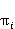) = log (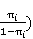(1)

Taking the antilog of the equation (1), one derives an equation that can be used in the possibility of the occurrence of an event as follows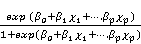(2)

Where π is the probability of the outcome of interest or the event. The model will be used to predict the likelihood of default.

2.2. Parameter Estimation

The regression coefficients are estimated by the method of maximum likelihood method.

2.3. Maximum Likelihood Method for Logistic Regression

Since each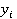represents a binominal count in the ith population the joint probability function of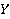is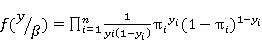(3)

The ML estimation is the values of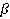that maximizes the likelihood function of equation (3) After rearranging the terms the equation to be maximized can be written as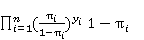(4)

The logistic regression model equates the logit transform to the log odds of the probability of success.(5)

Exponentiating both sides we get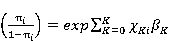(6)

Solving for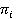we get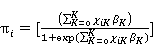(7)

Substituting equation 2.5 and 2.7 for the second term, equation 2.4 becomes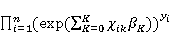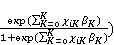(8)

Simplifying the first product equation 2.8 becomes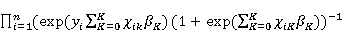(9)

This is the kernel of the likelihood function to maximize. This can be simplified by taking logs so as to differentiate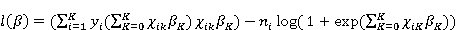(10)

To obtain the critical points of the log likelihood function, set the first derivate on eachequal to zero. Differentiating equation (10) we get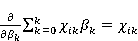(11)

Since the other term in the summation does not depend on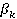they can be treated as constants. Differentiating the second half of equation 2.10 we get.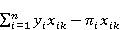(12)

The maximum likelihood estimates forcan be found by setting each of the K+1 equations in equation (12) to zero and solving for each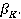Setting the equation (12) equal to zero results in a system of K+1 nonlinear equations each with K+1 unknown variables. To solve this system of nonlinear equations we can either use Newton- Raphson method of Fishers scoring method.

2.4. Extreme Value Theory

Extreme value theory is a robust framework to analyze the tail behavior of distributions Embrechts et al 

The class of GEV regression distributions they are flexible with the tail-shape parameter controlling the shape and size of the tails of the three different families of distributions nested under it.

The three families can be nested into a single parametric representation as shown by Jenkinson 

2.4.1. Generalized Extreme Value Distribution

Generalized extreme value distribution (GEV) is a family of continuous probability distribution developed within extreme value theory to combine the Gumbel, Frechet and Weibull also known as type I, II and III extreme value distributions.

The generalized extreme value distribution has cumulative distribution function given by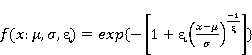(13)

For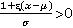where µϵ is the location parameter, σ˃0 the scale parameter and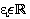the shape parameter that governs the tail behavior of the limiting distribution.

The probability density function is given by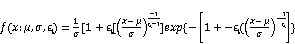(14)

AgainThe GEV distribution encompasses the three types of limiting distribution Gnedenko (1943)

Type 1: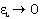the Gumbel family

The cdf is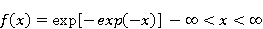(15)

Type II: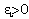the Frechet family.

The cdf is given by(16)

Type III: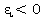the Weibull family

The CDF is given by(17)

However, the parameter µ is not the mean but does represent the Centre of the distribution, and the scale parameter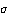is not the standard deviation but does govern the size of the deviations about µ.

To estimate the PD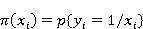(18)

The response curve for the GEV is given by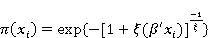(19)

The link function of the GEV model is given by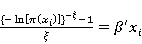(20)

That represents a non-canonical link function

For the interpretation of the parameters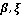we suppose that the value of the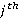regressor (with j=1………k) is increased by one unit and, all other variables remain unchanged.

2.4.2. Parameter Estimation of the GEV

The GEV model contains three parameters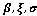. These parameters can be estimated using either parametric or non-parametric methods.

In parametric methods, we are going to discuss the maximum likelihood method

2.4.3. Maximum Likelihood Estimation of a GEV Model

Let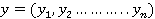be a simple random sample of size n from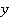the log-likelihood function is given by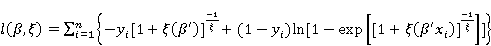(21)

For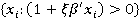The score functions are given by are obtained by differentiating the log likelihood function on the known parameters ξ and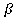.(22)

For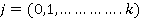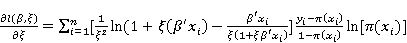(23)

The MLE of the parameters ξare dependent and they cannot be computed separately. The score functions do not have closed form, the MLE need to be obtained by numerically maximizing the log-likelihood function using iterative optimization algorithms.

Case 1: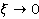For initial estimate for ξ a value close to zero, our GEV model becomes the Gumbel regression model with response curve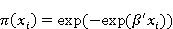(24)

The log-likelihood function of the Gumbel regression is given by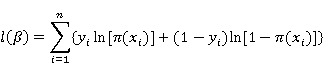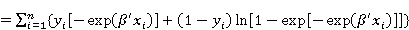(25)

The score function is given by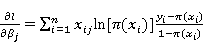For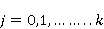(26)

To identify the initial values forwe choose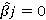for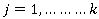by substitutingfor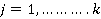in equation 2.26 we obtain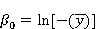(27)

Afterward by substituting the initial values for the parameter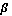in equation 2.24 we obtain the estimate of ξ for the first step of the relative procedure. By using this estimate of ξ in equation 2.23 we obtain the estimates of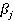with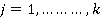for the first step in GEV regression.

Case II: when ξ<0

The GEV becomes the Weibull regression model; the cumulative distribution is given by(28)

For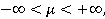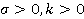Where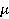and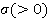are, respectively, a location and a scale parameters andis a shape parameter.

The response curve for Weibull is given by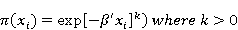(29)

The response curve of the Weibull regression model is a particular case of the GEV response curve for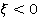.

The link function of the Weibull regression model is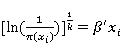(30)

The log-likelihood of the Weibull regression is given by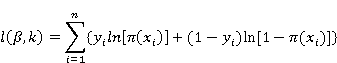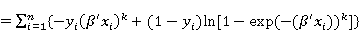(31)

The score functions are given by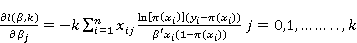(32)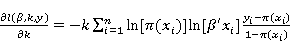(33)

To apply an iterative algorithm, the initial values of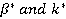for the parameters should be identified. If take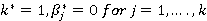and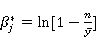(34)

We obtain the initial value (35) by substituting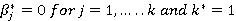in equation 2.34.

3. Results and Discussions

The empirical data analysis is based on set of data for the year 2007 for 5000 applicants whose loans were approved in one of the Kenya Commercial banks (Backlys Bank of Kenya)

Fitting the regression model

Estimating the probability of default using the logistic regression model, the factors under study were age, gender, job category, the level of education, level of income, debt income and marital status. The variable of interest was loan status which was code as 1 for defaulters and 0 for non- defaulters

Significance of Predictor Variables

At 5% of significance, the study found out that intercept, age, employment category, the level of income and the debt income were statistically significant.

Table 1. Regression Coefficients.

 Estimate Std Error Z value Pr(>|z|) Intercept -1.6209 0.3686 -4.40 0.0000 gender -0.0284 0.1171 -0.24 0.8084 age -0.0408 0.0068 -6.01 0.0000 ed 0.0243 0.0203 1.19 0.2322 jobcat -0.0195 0.0391 -0.50 0.6176 empcat -0.0331 0.0880 -3.77 0.0002 income 0.0051 0.0011 4.65 0.0000 debtinc 0.0844 0.0078 10.83 0.0000 marital -0.1148 0.1175 -0.98 0.3283

If the estimated regression coefficient of a variable is positive, an increase in the value will lead to an increase in the estimated PD, holding all other variables constant. When the coefficient is negative an increase in its value will produce a corresponding decrease in the estimated PD

The regression coefficients found significant were used to build the logistic regression model, which can be used to estimate the probability of default.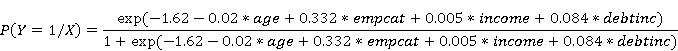The above model can be used to estimate the probability of default of the customers likely to default loan. The null deviance of this model is 2541.6

Dropping the intercept again we obtain the factors that are significant as shown in Table 2

Table 2. Regression Coefficients.

 Estimate Std Error Z value Pr(>|z|) gender -0.0825 0.1154 -0.72 0.4743 age -0.0470 0.0067 -7.03 0.0000 edu 0.0476 0.0123 -3.87 0.0001 jobcat -0.0681 0.0378 -1.80 0.0719 empcat -0.3738 0.0884 -4.23 0.0000 income 0.0066 0.0011 5.76 0.0000 debtinc 0.0775 0.0077 10.06 0.0000 marital -0.2043 0.1149 -1.78 0.0752

For the second model, five factors are significant but this time level of education is significant together with age, employment category, the level of income and debt income.

The regression coefficients found significant were used to build the logistic regression model which can be used to estimate the probability of default.This model can again be used to estimate the probability of default of the customers likely to default loan. The null deviance of this model is 6931.5

This model is a better fit than the first model since it has higher null deviance.

Finally, we can construct a model with the significant factors. We obtain the following regression coefficients in Table 3

Table 3. Regression Coefficients.

 Estimate Std Error Z value Pr(>|z|) age -0.0442 0.0063 -7.002 0.0000 edu -0.0647 0.0103 -6.241 0.0001 empcat -0.4506 0.0790 -5.700 0.0000 income 0.0069 0.0011 6.122 0.0000 debtinc 0.0764 0.0076 9.943 0.0000

All the regression factors are significant, and there is no change in null devianceAgain this equation can be used to predict the probability default.

Fitting the GEV regression model

When the percentages of defaulters are very low, the defaulters’ characteristics are more informative than those of non-defaulters. Therefore, defaulters’ features can be better represented by the tail of the response curve for the values close to 1 which can be modeled using the GEV regression model.

Significance of the predictor variables

At 5% of significance, the study found out that intercept, age, employment category, level of income and debt income were statistically significant as shown in Table 4

Table 4. Regression Coefficients.

 Estimate Std Error Z value Pr(>|z|) Intercept -0.6374 0.1720 -3.704 0.0000 gender -0.0121 0.0557 -0.218 0.8277 age -0.0185 0.0029 -6.260 0.0000 ed 0.0089 0.0094 0.945 0.3446 jobcat -0.0072 0.0186 -0.389 0.6972 empcat -0.1764 0.0395 -4.458 0.0000 income 0.0027 0.0005 5.524 0.0000 debtinc 0.0447 0.0039 11.189 0.0000 marital -0.0656 0.0558 -1.174 0.2405

The value of ξ=-0.25 which means that ξ< 0, this becomes the Weibull distribution which is a particular case of GEV distribution. Using the significant factors, we can build a model which will predict the probability of default using the equation belowDropping the intercept again we obtain the factors that are significant as shown in Table 5

Table 5. Regression Coefficients.

 Estimate Std Error Z value Pr(>|z|) gender -0.0422 0.0551 -0.767 0.4432 age -0.0209 0.0029 -7.206 0.0000 edu -0.0188 0.0057 -3.293 0.0001 jobcat -0.0269 0.0179 -1.502 0.1332 empcat -0.1916 0.0884 -4.858 0.0000 income 0.0032 0.0004 6.803 0.0000 debtinc 0.0775 0.0039 10.74 0.0000 marital -0.0985 0.0551 -1.78 0.0740

Under this model age, the level of education, employment category, the level of income, debt income is statistically significant just like in logistic regression model. We use them to build another model which can be used to estimate the probability of defaultPredictive accuracy

For financial institutions the underestimation of the probability of default could be very risky. The objective of this section shows that the GEV model overcomes the drawbacks of the logistic model in the modeling of rare events. To avoid over-fitting, data is randomly divided into two parts a sample on which the regression models are estimated and control sample on which we evaluate the predictive accuracy of the models. For comparing the models, the study used the confusion matrix which was later used to tabulate the predictive accuracy of the model.

The predictive accuracy of both models is tabulated in Table 6

Table 6. Average forecasting accuracy for different PDs on the sample.

 Sample percentage Of defaulters Models GEV regression accuracy Logistic regression Accuracy 0.1 0.825(82.5%) 0.814(81.4%) 0.05 0.834(83.4%) 0.804(80.4%) 0.025 0.836(83.6%) 0.802(80.2%) 0.01 0.876(87.6%) 0.794(79.4%) 0.005 0.901(90.1%) 0.793(79.3%)

From the table 5, it shows the predictive accuracy of both models that is GEV regression model and the logistic regression model. The GEV model accuracy improves its predictive accuracy by reducing the sample percentage of defaulters while the logistic regression model becomes worse as the defaulters reduce. The Predictive accuracy of the GEV model is always higher than the predictive accuracy of the logistic regression.

4. Conclusion

The main objective of the study was to come up with a regression model that could overcome the drawbacks of the logistic regression model in underestimating the PD on loans. Since lending is a risky venture, its good for financial institutions to take caution by being able to identify the probability of default, GEV is a suitable function for modeling extreme and rare events. The GEV distribution depends on the regression parameters and the shape parameter of the GEV distribution. The main advantage of the GEV model is the good performance in identifying defaults for this characteristic the drawback of the logistic regression model of underestimating the PD is overcome. It is much more costly to classify a defaulter as a non-defaulter when he is a defaulter. In particular, when a defaulter is categorised as a non-defaulter by the model, banks will give a loan. If the borrower becomes a defaulter, the bank may lose the whole part of the credit exposure. On the contrary, when a non-defaulter is categorised as defaulted, the banks lose interest on loans only. For this reason, the identification of defaulters is very important objective for the bank. By reducing the sample percentage of defaults, the predictive performance of the logistic regression to identify defaults becomes poorer. On the contrary, the accuracy of the GEV model to identify defaults improves with reduction the sample percentage of default.

Recommendation

Banks and financial institutions could improve their assessment and efficiency by using GEV models to predict the probability of default. The study results found out that the GEV model performs better than the logistic regression model for rare events. More studies can be carried out using this model in estimating the probability of bank default and establish the reasons of collapsing of banks in Kenya economy. Further studies can be done on the same work using the GEV link function in a generalized additive model.

References

1. Andrew, C. (2004).Basel II: The reviewed framework of June 2004.Geneva, Switzerland.
2. Agresti, A. (2002). An introduction to categorical data analysis. New York: Wiley.
3. Anatoly B.J (2014). The probability of default models of Russian banks. Journal of Institute of Economics in Transition 21(5),203-278.
4. Adrea Ruth.(2010). Measuring the likelihood of small Business default; Journal of Applied Sciences 33(7),1289-1386.
5. Altman E.(1968). Financial ratios, discriminant analysis, and prediction of corporate bankruptcy. Journal of Finance 23(4)589-609.
6. Alexander B.(2012) Determinant of bank failures thecase of Russia, Journal of Applied Statistics,78(32),235-403.
7. Beirlant, (2004). Statistics of extremes. Hoboken, NJ: Wiley.
8. Calabrese, R. (2012). Modelling SME loan defaults as rare events: The generalized extreme value regression.Journal Of Applied Statistics, 00(00), 1-17.
9. Calabrese R. (2011).Generalizedextreme value regression for binary rare events data: an application to credit default. Journal of Applied Statistics, 2(4), 4-8.
10. Castillo, E. (2005). Extreme value and related models with applications in engineering and science. Hoboken, N.J.: Wiley.
11. Coles, S. (2001). An introduction to statistical modeling of extreme values. London: Springer.
12. David (1977). Early warning of bank failure: A logit regression approach. Journal of Banking and Finance, 19, 109-301.
13. Dobson A.J (2002). An Introduction to Generalized linear models. 2nd ed. Boca rayon.
14. Eliason, S.R (1993) Maximum Likelihood Estimation: Logic and Practice. Sage University Paper series on Quantitative Application in social sciences, series no.07-096.Newbury Park.
15. Falk, M., HuÌˆsler, J. & Reiss,R. (1994).Laws of small numbers: extremes and rare events;[based on lectures given at the DMV Seminar on "Laws of small numbers; extremes and rare events," held at the Katholische UniversitaÌˆtEichstaÌˆtt from October 20 - 27, 1991]. Basel [u.a.]: BirkhaÌˆuser.
16. Galambos, J. (1978). The asymptotic theory of extreme order statistics. New York: Wiley.
17. Gilli, M., &KeÌˆllezi, E. (2000).Extreme value theory for tail-related risk measures. Geneva: FAME.
18. Goodhart, C. (2011). The Basel Committee on Banking Supervision. Cambridge: Cambridge University Press.
19. Gumbel, E. (1958). Statistics of extremes. New York: Columbia University Press.
20. Haan, L., & Ferreira, A. (2006).Extreme value theory. New York: Springer.
21. Haotian chen and Ziyuan Chen. Data mining on loan Default prediction. Journal of Institute of Economics in Transition 214(7),256-298.
22. Jenkison,(1956). Statistics of extremes. Hoboken, NJ: Wiley.
23. Junjie Liang(2013) Predicting borrowers chance of defaulting on credit loans. American Journal of Theoretical and Applied Statistics,1345(2),4556-4598.
24. Leadbetter, M., Lindgren, G., &RootzeÌn, H. (1983).Extremes and related properties of random sequences and processes. New York: Springer-Verlag.
25. Leadbetter, M., Lindgren, G., &Rootzen, H. (1980).Extremal and Related Properties of Stationary Processes. Part II. Extreme Values in Continuous Time. Ft. Belvoir: Defense Technical Information Center.
26. Lenntand Golet(2014). Symmetric and asymmetric binary choice models for corporate bankruptcy, Journal of social and behavior sciences,124(14),282-291.
27. McCullagh P., Nelder J.A (1989) Generalized linear model, Chapman Hall, Newyork.
28. O.Adem.,&Waititu, A. (2012). Parametric modeling of the probability of bank loan default in Kenya. Journal of Applied Statistics, 14(1), 61-74.
29. Oliveira, J. (1984). Statistical Extremes and Applications. Dordrecht:Springer Netherlands.
30. Omkar G.(2002).Predicting loan defaults. American Journal of Theoretical and Applied Statistics,15(3),3543-3789.
31. Rafaella, C.Giampiero, M. Bankruptcy Prediction of small and medium enterprises using s flexible binary GEV extreme value model. American Journal of Theoretical and Applied Statistics,1307 (2),3556-3798.
32. Paul Embrechts, Resnick, Sydney. (1987). Extreme values, regular variation, and point processes. New York: Springer-Verlag.
33. Semmes, T. (2011).Gumbel. Newyork.
34. Sjur Westgaard (2002). Capital Structure and the prediction of bankruptcy.American Journal of applied statistics,45(57), 543-678.
35. Singhee, A., &Rutenbar, R. (2010).Extreme statistics in nanoscale memory design. New York: Springer.
36. Uday Rajan(2010).Statistical models and incentives, Journal of Applied Sciences,100(2)3456-3500
37. Von Mises, (1936). Theory of Statistics of extremes. Hoboken, NJ: Wiley.
38. Wikipedia,(2015).Generalized extreme value distribution. Retrieved 2 December 2015, from http://en.wikipedia.org/wiki/Extreme_value_distribution

 Contents 1. 1.1. 1.2. 1.3. 1.4. 1.5. 2. 2.1. 2.2. 2.3. 2.4. 3. 4.
Article ToolsAbstractPDF(256K)